# Correction factors in `shorts` for estimation using split times

To explain the use of correction factors in `shorts` package functions, let’s consider the following example. Imagine we have an athlete, John, with the following true short sprint characteristics: maximum sprinting speed (MSS) of 8.5\(m/s\) and maximum acceleration (MAC) of 7.5 \(m/s^2\). Since TAU (or relative acceleration) is equal to \(MSS \;/\; MAC\), in this case that is 1.133. Let’s generate John’s sprint kinematics during 40m sprint from his known and true characteristics:

``````library(shorts)
library(tidyverse)

set.seed(1667)

john_MSS <- 8.5
john_MAC <- 7.5
john_TAU <- john_MSS / john_MAC

john_kinematics <- tibble(
name = "John",
distance = seq(0, 40, length.out = 10^4),
time = shorts::predict_time_at_distance(distance, john_MSS, john_TAU),
velocity = shorts::predict_velocity_at_distance(distance, john_MSS, john_TAU),
acceleration = shorts::predict_acceleration_at_distance(distance, john_MSS, john_TAU),
power = velocity * acceleration
)``````

Now we can plot this against time and distance. Let’s plot against distance first:

``````john_kinematics_per_distance <- john_kinematics %>%
gather("metric", "value", -name, -distance)

ggplot(john_kinematics_per_distance, aes(x = distance, y = value, color = name)) +
theme_minimal() +
geom_line(alpha = 0.8) +
facet_wrap(~metric, scales = "free_y") +
ylab(NULL)``````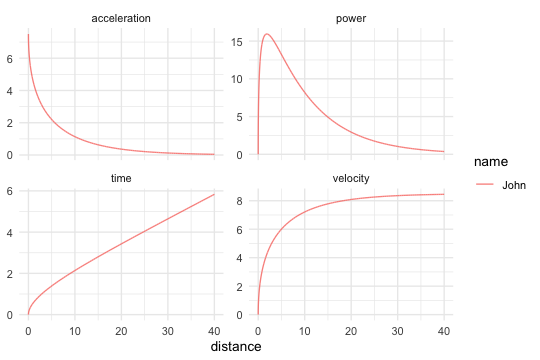And finally against time:

``````john_kinematics_per_time <- john_kinematics %>%
gather("metric", "value", -name, -time)

ggplot(john_kinematics_per_time, aes(x = time, y = value, color = name)) +
theme_minimal() +
geom_line(alpha = 0.8) +
facet_wrap(~metric, scales = "free_y") +
ylab(NULL)``````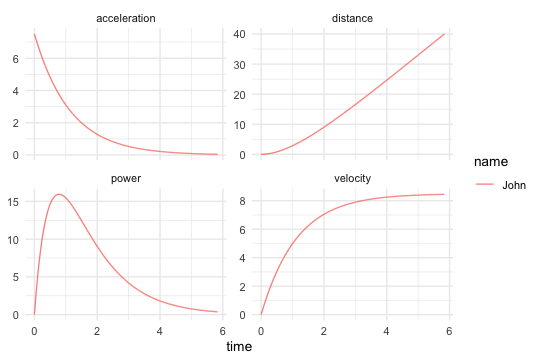Now, imagine John has an evil twin brother Jack (hint: this is counter-factual reasoning often implemented in theory of measurement since it is damn hard to repeat everything under exactly the same conditions, unless of course, we talk about simulation as in here). Jack is completely the same as John (in terms of his sprint characteristics), but he plays soccer and that makes him a bit sneaky. So, Jack, sneaky as he is, decided to move slightly behind the first timing game (the gate at 0m which is responsible to starting the timer) and use body rocking motion. John, on the other hand, being a good twin brother as he is, perform starts in completely stand-still manner and exactly at the first timing gate (we have seen his sprint kinematics). This scenario is depicted on the figure below: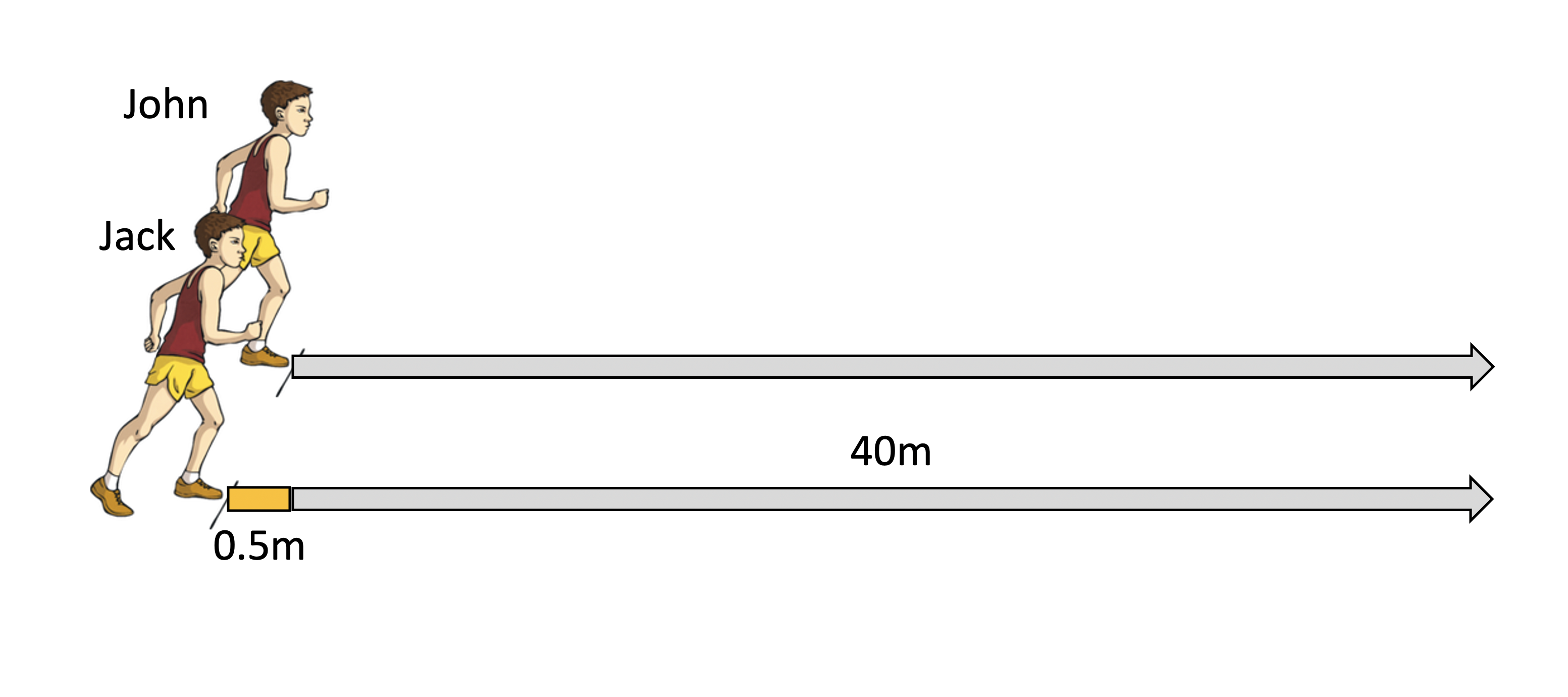Let’s see what will happen with Jack’s kinematics. Since they are equal, we just need to deduct distance and time it take to cover those 0.5m that Jack used:

``````jack_kinematics <- john_kinematics

jack_kinematics <- jack_kinematics %>%
# Remove those first 0.5m
filter(distance >= 0.5) %>%

# Now deduct time and distance
mutate(
distance = distance - min(distance),
time = time - min(time),

# And rename the athlete
name = "Jack"
)``````

Now we can compare them by merging the two data frames and plot kinematics per distance and per time:

``````sprint_kinematics <- rbind(
john_kinematics,
jack_kinematics
)

sprint_kinematics\$name <- factor(
sprint_kinematics\$name,
levels = c("John", "Jack")
)``````
``````kinematics_per_distance <- sprint_kinematics %>%
gather("metric", "value", -name, -distance)

ggplot(kinematics_per_distance, aes(x = distance, y = value, color = name)) +
theme_minimal() +
geom_line(alpha = 0.8) +
facet_wrap(~metric, scales = "free_y") +
ylab(NULL)``````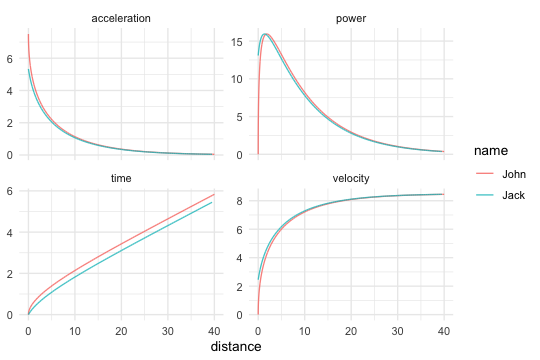``````kinematics_per_time <- sprint_kinematics %>%
gather("metric", "value", -name, -time)

ggplot(kinematics_per_time, aes(x = time, y = value, color = name)) +
theme_minimal() +
geom_line(alpha = 0.8) +
facet_wrap(~metric, scales = "free_y") +
ylab(NULL)``````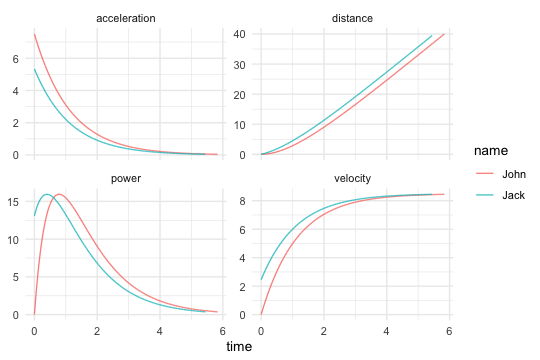The differences look obvious since we have the power of this theoretical simulation, but in the real life, we often use distances at 5, 10, 20, 30 and/or 40m and this cheating (or better yet, different starting position) cannot be seen so easily.

So let’s rerun this, but using the common sprint distances of 5, 10, 20, 30, and 40m. To generate data for Jack, we need to add 0.5m to sprint distances, calculate times, and deduct time it take to cover first 0.5m that doesn’t trigger timing:

``````sprint_distance <- c(5, 10, 20, 30, 40)

john_split_kinematics <- tibble(
name = "John",
distance = sprint_distance,
time = shorts::predict_time_at_distance(distance, john_MSS, john_TAU)
)

john_split_kinematics
#> # A tibble: 5 x 3
#>   name  distance     time
#>   <chr>    <dbl> <I<dbl>>
#> 1 John         5     1.39
#> 2 John        10     2.14
#> 3 John        20     3.43
#> 4 John        30     4.64
#> 5 John        40     5.83

jack_split_kinematics <- tibble(
name = "Jack",
distance = sprint_distance,
true_distance = distance + 0.5,
true_time = shorts::predict_time_at_distance(true_distance, john_MSS, john_TAU),
`time_05m` = shorts::predict_time_at_distance(0.5, john_MSS, john_TAU),
time = true_time - `time_05m`
)

jack_split_kinematics
#> # A tibble: 5 x 6
#>   name  distance true_distance true_time time_05m     time
#>   <chr>    <dbl>         <dbl>  <I<dbl>> <I<dbl>> <I<dbl>>
#> 1 Jack         5           5.5      1.47    0.386     1.08
#> 2 Jack        10          10.5      2.21    0.386     1.82
#> 3 Jack        20          20.5      3.49    0.386     3.11
#> 4 Jack        30          30.5      4.70    0.386     4.32
#> 5 Jack        40          40.5      5.89    0.386     5.51``````

Let’s see how this cheating affects estimated sprint parameters. This is very useful exercises since we know the true parameters, and now we can see how they differ:

``````# Since this is a perfect simulation and stats::nls will complain
# we need to add very small noise, or measurement error
john_split_kinematics\$time <- john_split_kinematics\$time + rnorm(length(sprint_distance), 0, 10^-5)
jack_split_kinematics\$time <- jack_split_kinematics\$time + rnorm(length(sprint_distance), 0, 10^-5)

john_split_params <- with(
john_split_kinematics,
shorts::model_using_splits(distance, time)
)

jack_split_params <- with(
jack_split_kinematics,
shorts::model_using_splits(distance, time)
)

split_parameters <- rbind(
unlist(john_split_params\$parameters),
unlist(jack_split_params\$parameters)
)

rownames(split_parameters) <- c("John", "Jack")

round(split_parameters, 2)
#>       MSS  TAU   MAC  PMAX time_correction distance_correction
#> John 8.50 1.13  7.50 15.94               0                   0
#> Jack 8.14 0.62 13.15 26.77               0                   0``````

As can be seen from the output above, estimated sprint parameters are different. Particularly large difference can be seen for TAU, MAC, and PMAX. Let’s see the difference in model fit (please note that we have added very small noise to the data to allow for the model to be fitted):

``````split_model_fit <- rbind(
unlist(john_split_params\$model_fit),
unlist(jack_split_params\$model_fit)
)

rownames(split_model_fit) <- c("John", "Jack")

round(split_model_fit, 3)
#>        RSE R_squared minErr maxErr maxAbsErr RMSE   MAE  MAPE
#> John 0.000         1  0.000  0.000     0.000 0.00 0.000 0.000
#> Jack 0.039         1 -0.037  0.049     0.049 0.03 0.027 1.378``````

As expected, perfect model fit for John (since we generated his performance from the model itself), and some error involved with Jack. Let’s see visually these differences (assuming they both had the same proper start):

``````john_split_kinematics\$predicted_time <- shorts::predict_time_at_distance(
sprint_distance,
john_split_params\$parameters\$MSS,
john_split_params\$parameters\$TAU
)

jack_split_kinematics\$predicted_time <- shorts::predict_time_at_distance(
sprint_distance,
jack_split_params\$parameters\$MSS,
jack_split_params\$parameters\$TAU
)

split_kinematics <- rbind(
john_split_kinematics,
select(jack_split_kinematics, name, distance, time, predicted_time)
)

split_kinematics\$name <- factor(
split_kinematics\$name,
levels = c("John", "Jack")
)

split_kinematics\$difference <- with(
split_kinematics,
predicted_time - time
)

ggplot(split_kinematics, aes(x = distance, y = difference, color = name)) +
theme_minimal() +
geom_point(alpha = 0.8) +
geom_line(alpha = 0.8) +
ylab("Predicted - observed")``````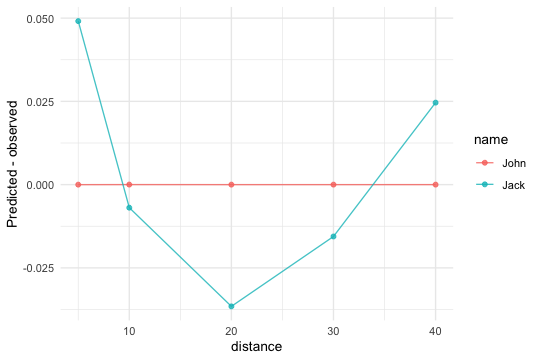As can be seen from the figure above, at particular distance the residuals (the difference between model prediction and observation) are different. This is because the model is ill defined assuming no cheating for Jack.

But how do we fix this? There are few options. In ideal scenario, we should know the distance shift (i.e., 0.5m in our case) and time it takes to cover it. This of course depends on the true parameters (given the model) and it is something we cannot know in real life. Other option is to use correction factor, in a simple form of `time_correction` which we simply add to split times (Haugen, Tønnessen, and Seiler 2012; Haugen, Breitschädel, and Seiler 2019). `time_correction` is usually around 0.15 - 0.3seconds, but this depends on the type of the start, acceleration abilities of the athletes and so forth. It does represent a good and useful rule of a thumb.

If you look at Jack split kinematics table, you can notice the time it takes to cover that 0.5m (`time_05m` in the table). This is similar to `time_correction`, but it is not the same, since we have deducted known distance of 0.5 as well. Let’s use simple heuristic, of 0.3seconds that needs to be added to out split times. Function `shorts::model_using_splits` allows for using such a correction factor:

``````jack_params_with_correction <- with(
jack_split_kinematics,
shorts::model_using_splits(distance, time, time_correction = 0.3)
)

unlist(jack_params_with_correction\$parameters)
#>                 MSS                 TAU                 MAC                PMAX
#>            8.522139            1.116450            7.633248           16.262899
#>     time_correction distance_correction
#>            0.300000            0.000000``````

As can be seen, this is much better (very close to John’s profile). Here are the model fit metrics:

``````unlist(jack_params_with_correction\$model_fit)
#>          RSE    R_squared       minErr       maxErr    maxAbsErr         RMSE
#>  0.004669167  0.999995356 -0.006676947  0.003387867  0.006676947  0.003616722
#>          MAE         MAPE
#>  0.003033239  0.179724847``````

Let’s check the prediction residuals now:

``````jack_split_kinematics_corrected <- select(jack_split_kinematics, name, distance, time)

jack_split_kinematics_corrected\$predicted_time <- shorts::predict_time_at_distance(
sprint_distance,
jack_params_with_correction\$parameters\$MSS,
jack_params_with_correction\$parameters\$TAU,
time_correction = 0.3
)

jack_split_kinematics_corrected\$difference <- with(
jack_split_kinematics_corrected,
predicted_time - time
)

jack_split_kinematics_corrected\$name <- "Jack w/0.3s correction"

split_kinematics <- rbind(
split_kinematics,
jack_split_kinematics_corrected
)

ggplot(split_kinematics, aes(x = distance, y = difference, color = name)) +
theme_minimal() +
geom_point(alpha = 0.8) +
geom_line(alpha = 0.8) +
ylab("Predicted - observed")``````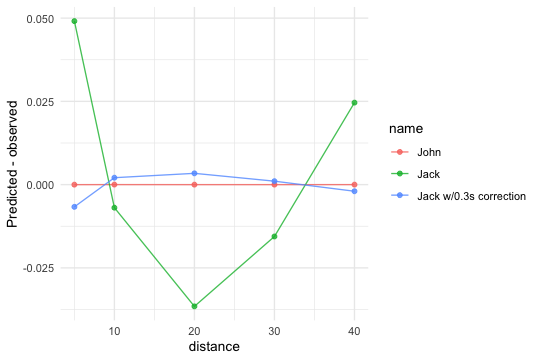Not only the estimated parameters (i.e., TAU and MSS) are much closer to true parameters, the model predictions are much better as indicated with the above graph.

Additionally, we can actually estimate `time_correction` form the observed split times, together with estimating MSS and TAU parameters (as we have done so far). The engine underneath the `shorts` package is `nls` function, that perform non-linear least squares regression. Rather than using `shorts::model_using_splits` function (which estimates two parameters: MSS and TAU), we can use `shorts::model_using_splits_with_time_correction` (which estimates three parameters: MSS, TAU, an time_correction):

``````jack_param_with_estimated_time_correction <- with(
jack_split_kinematics,
shorts::model_using_splits_with_time_correction(distance, time)
)

unlist(jack_param_with_estimated_time_correction\$parameters)
#>                 MSS                 TAU                 MAC                PMAX
#>           8.4736411           1.0627127           7.9735952          16.8913459
#>     time_correction distance_correction
#>           0.2720356           0.0000000``````

Here are the model fit metrics:

``````unlist(jack_param_with_estimated_time_correction\$model_fit)
#>           RSE     R_squared        minErr        maxErr     maxAbsErr
#>  0.0016131780  0.9999995979 -0.0009223064  0.0016968979  0.0016968979
#>          RMSE           MAE          MAPE
#>  0.0010202634  0.0009375994  0.0447416446``````

Let’s check the prediction residuals now:

``````jack_split_kinematics_corrected_est <- select(jack_split_kinematics, name, distance, time)

jack_split_kinematics_corrected_est\$predicted_time <- shorts::predict_time_at_distance(
sprint_distance,
jack_param_with_estimated_time_correction\$parameters\$MSS,
jack_param_with_estimated_time_correction\$parameters\$TAU,
jack_param_with_estimated_time_correction\$parameters\$time_correction
)

jack_split_kinematics_corrected_est\$difference <- with(
jack_split_kinematics_corrected_est,
predicted_time - time
)

jack_split_kinematics_corrected_est\$name <- "Jack w/est. correction"

split_kinematics <- rbind(
split_kinematics,
jack_split_kinematics_corrected_est
)

ggplot(split_kinematics, aes(x = distance, y = difference, color = name)) +
theme_minimal() +
geom_point(alpha = 0.8) +
geom_line(alpha = 0.8) +
ylab("Predicted - observed")``````